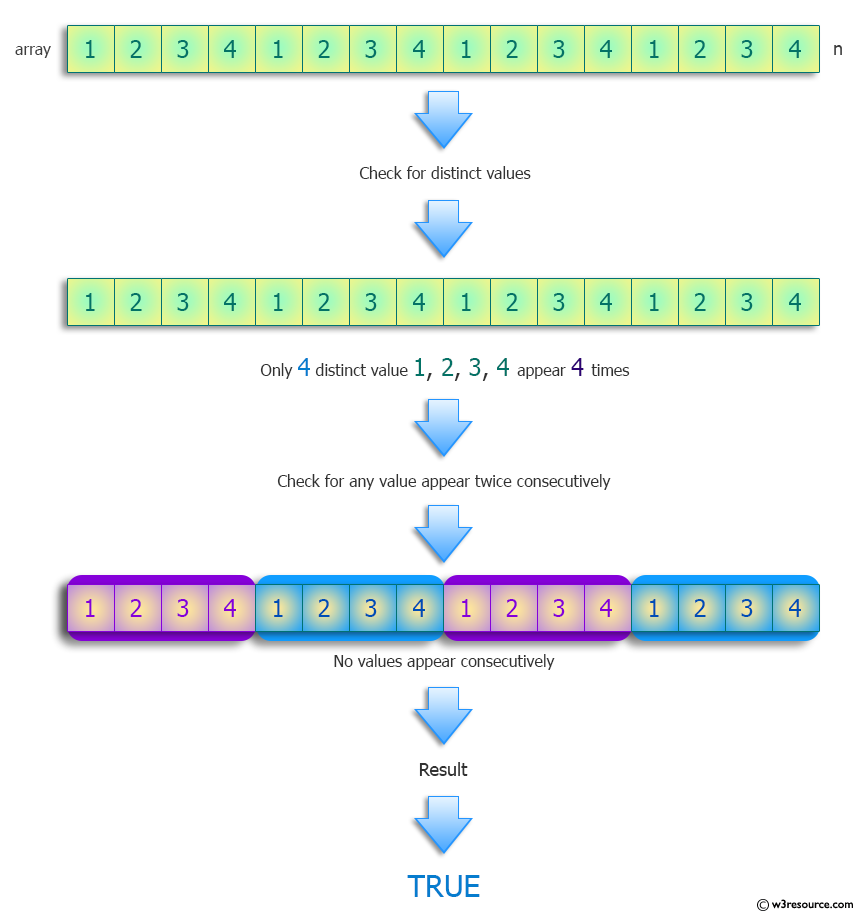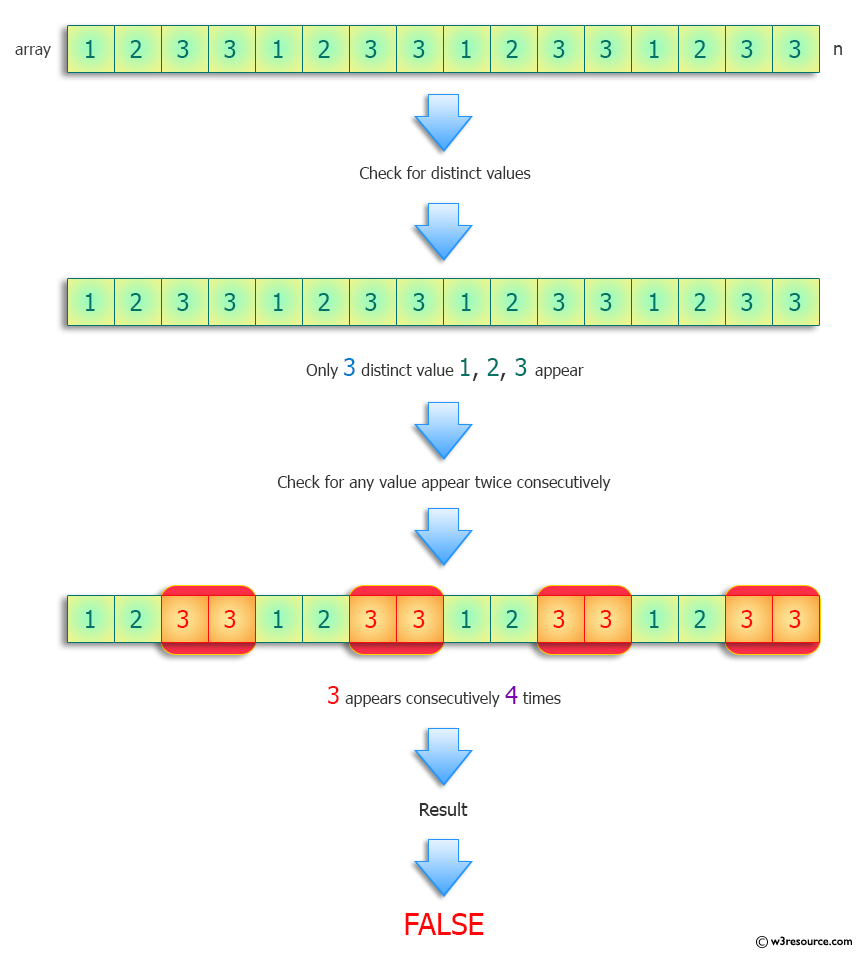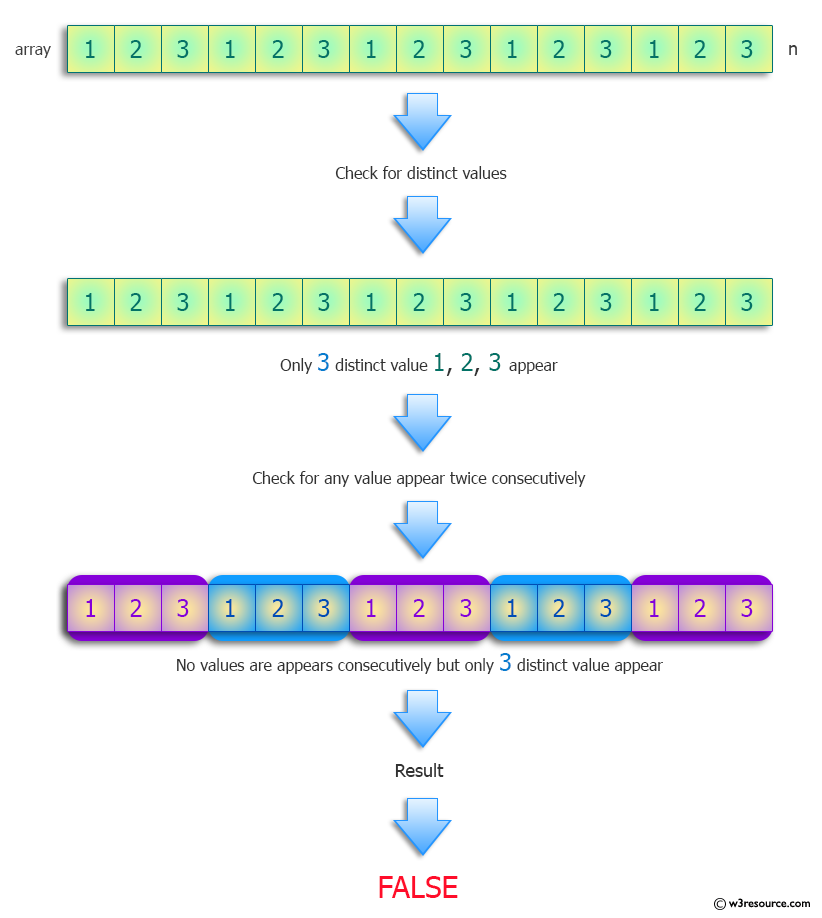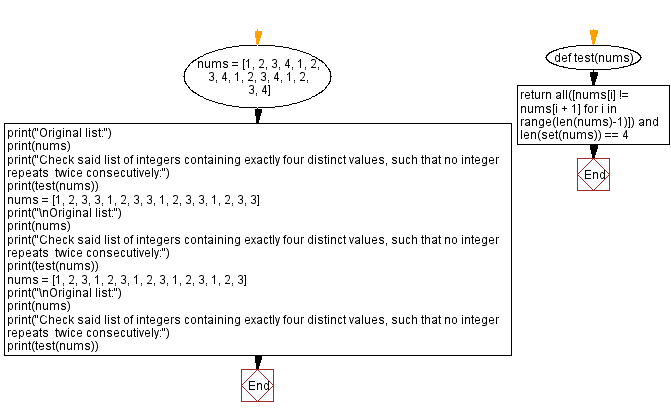﻿ Python: List integers containing exactly three distinct values, such that no integer repeats twice consecutively - w3resource# Python: List integers containing exactly three distinct values, such that no integer repeats twice consecutively

## Python Programming Puzzles: Exercise-9 with Solution

Write a Python program to find list integers containing exactly four distinct values, such that no integer repeats twice consecutively among the first twenty entries.

Note: The list needs to have length greater than ten.)

```Input:
[1, 2, 3, 4, 1, 2, 3, 4, 1, 2, 3, 4, 1, 2, 3, 4]
Output:
True

Input:
[1, 2, 3, 3, 1, 2, 3, 3, 1, 2, 3, 3, 1, 2, 3, 3]
Output:
False

Input:
[1, 2, 3, 1, 2, 3, 1, 2, 3, 1, 2, 3, 1, 2, 3]
Output:
False
```

Pictorial Presentation:Sample Solution:

Python Code:

``````#License: https://bit.ly/3oLErEI
def test(nums):
return all([nums[i] != nums[i + 1] for i in range(len(nums)-1)]) and len(set(nums)) == 4
nums = [1, 2, 3, 4, 1, 2, 3, 4, 1, 2, 3, 4, 1, 2, 3, 4]
print("Original list:")
print(nums)
print("Check said list of integers containing exactly four distinct values, such that no integer repeats  twice consecutively:")
print(test(nums))
nums = [1, 2, 3, 3, 1, 2, 3, 3, 1, 2, 3, 3, 1, 2, 3, 3]
print("\nOriginal list:")
print(nums)
print("Check said list of integers containing exactly four distinct values, such that no integer repeats  twice consecutively:")
print(test(nums))
nums = [1, 2, 3, 1, 2, 3, 1, 2, 3, 1, 2, 3, 1, 2, 3]
print("\nOriginal list:")
print(nums)
print("Check said list of integers containing exactly four distinct values, such that no integer repeats  twice consecutively:")
print(test(nums))
``````

Sample Output:

```Original list:
[1, 2, 3, 4, 1, 2, 3, 4, 1, 2, 3, 4, 1, 2, 3, 4]
Check said list of integers containing exactly four distinct values, such that no integer repeats  twice consecutively:
True

Original list:
[1, 2, 3, 3, 1, 2, 3, 3, 1, 2, 3, 3, 1, 2, 3, 3]
Check said list of integers containing exactly four distinct values, such that no integer repeats  twice consecutively:
False

Original list:
[1, 2, 3, 1, 2, 3, 1, 2, 3, 1, 2, 3, 1, 2, 3]
Check said list of integers containing exactly four distinct values, such that no integer repeats  twice consecutively:
False
```

Flowchart:## Visualize Python code execution:

The following tool visualize what the computer is doing step-by-step as it executes the said program:

Python Code Editor :

Have another way to solve this solution? Contribute your code (and comments) through Disqus.

What is the difficulty level of this exercise?

Test your Programming skills with w3resource's quiz.

﻿

## Python: Tips of the Day

Clamps num within the inclusive range specified by the boundary values x and y:

Example:

```def tips_clamp_num(num,x,y):
return max(min(num, max(x, y)), min(x, y))
print(tips_clamp_num(2, 4, 6))
print(tips_clamp_num(1, -1, -6))
```

Output:

```4
-1
```